# RD Sharma Solutions for Class 11 Chapter 16 - Permutations Exercise 16.1

This exercise discusses the problems based on the factorial (the continued product of first n natural numbers is called the “n factorial”). The solutions here are designed for students who wish to use RD Sharma textbook as a reference or guide to clarify their doubts pertaining to the problems and learn the correct steps in solving them. RD Sharma Class 11 Maths Solutions contains this exercise in the pdf format, which can be easily downloaded from the links given below.

## Download the pdf of RD Sharma Solutions for Class 11 Maths Exercise 16.1 Chapter 16 – Permutations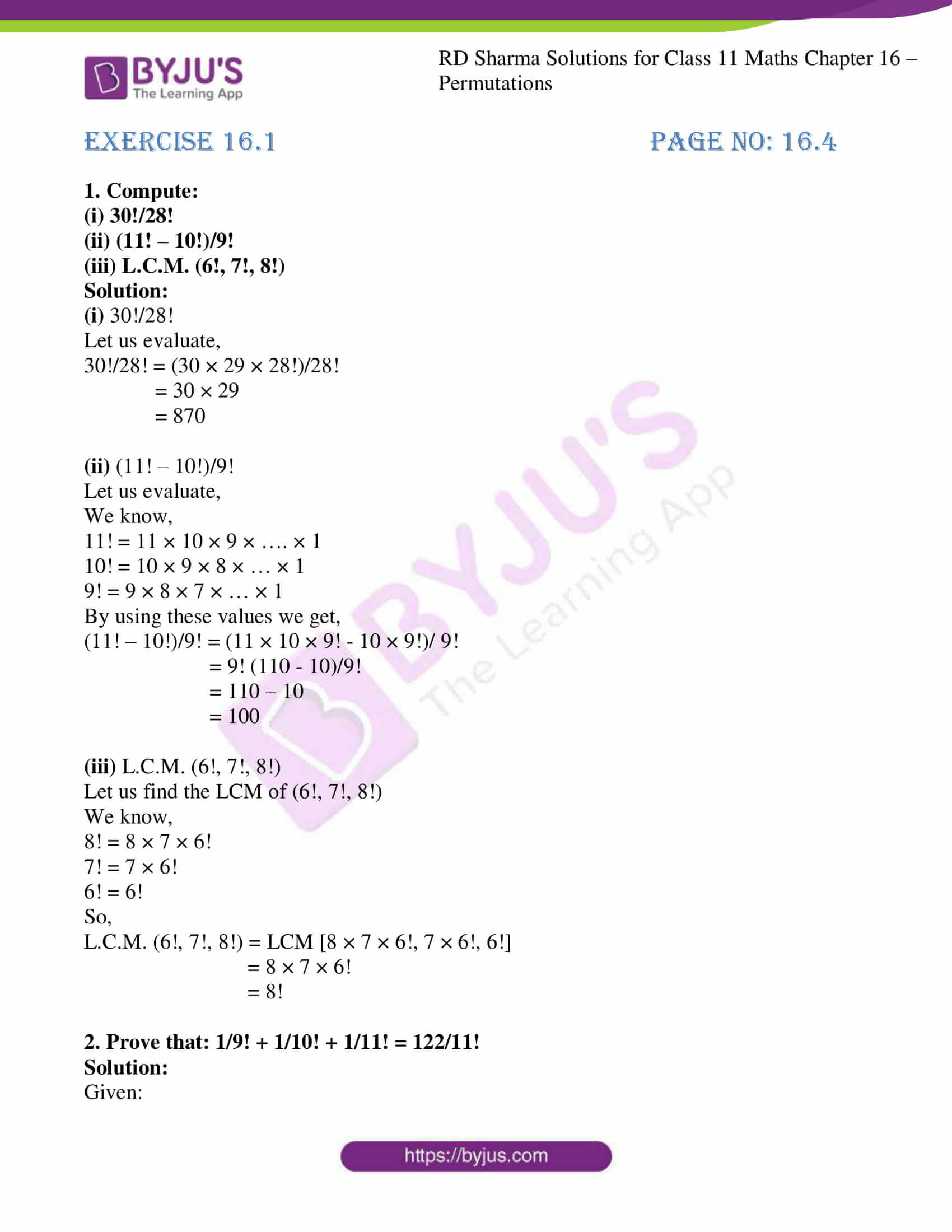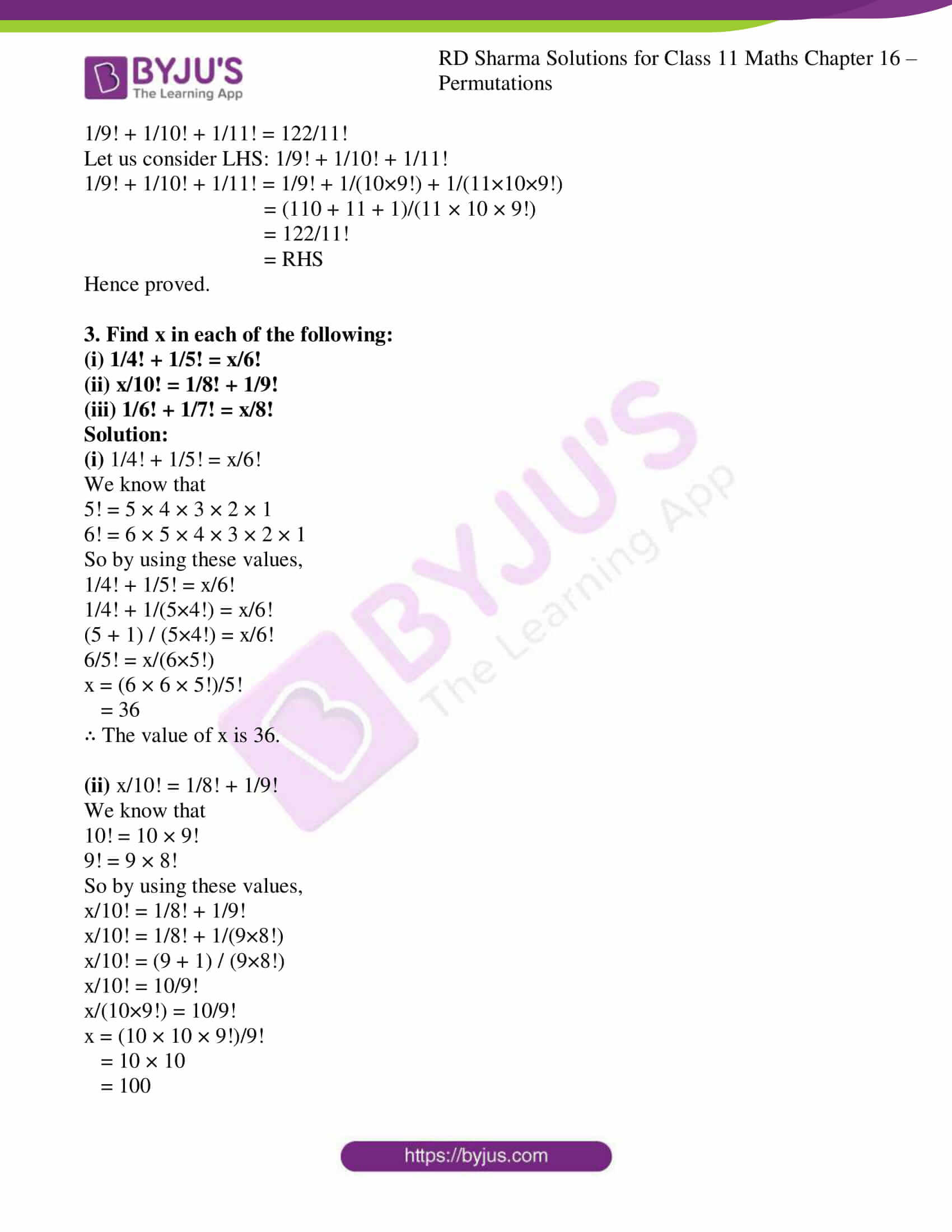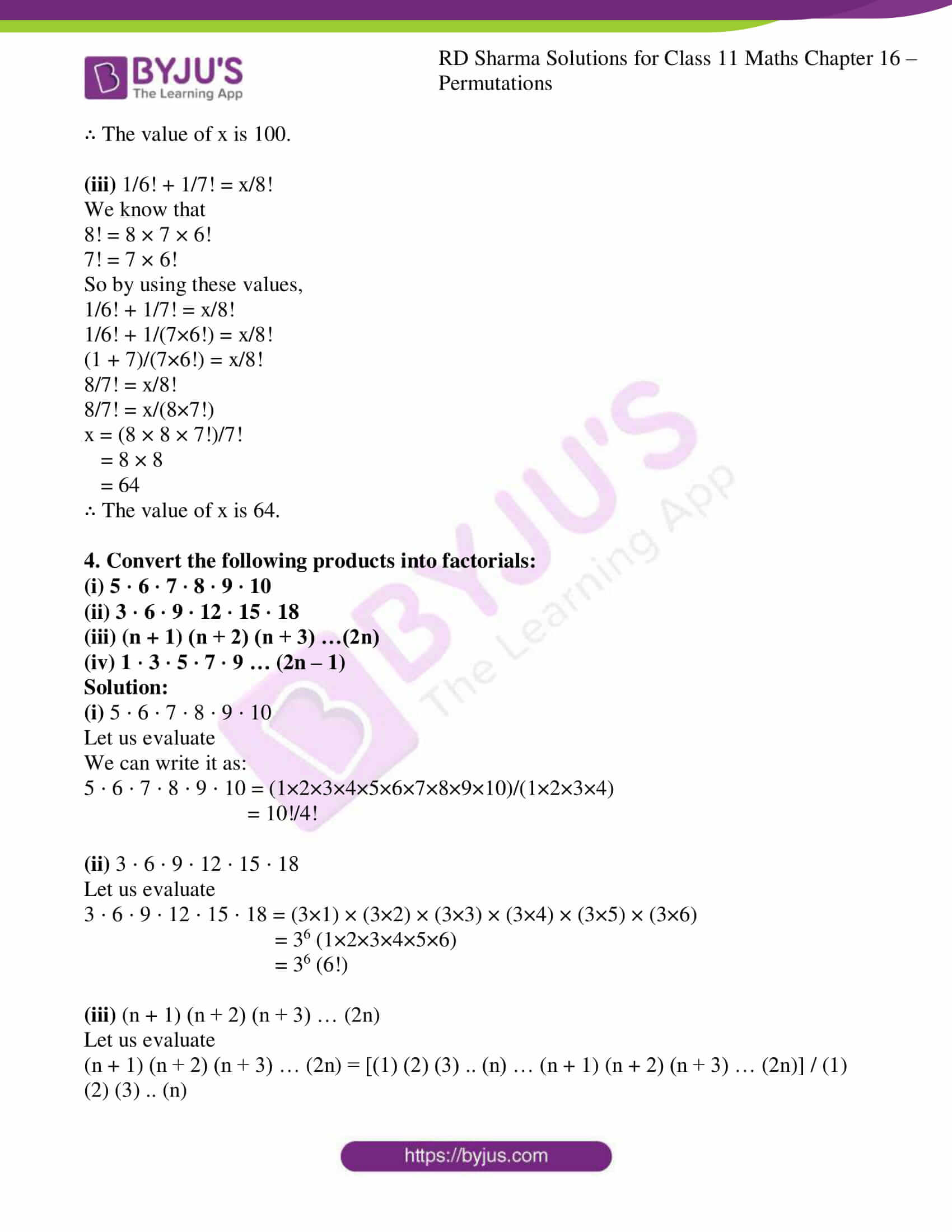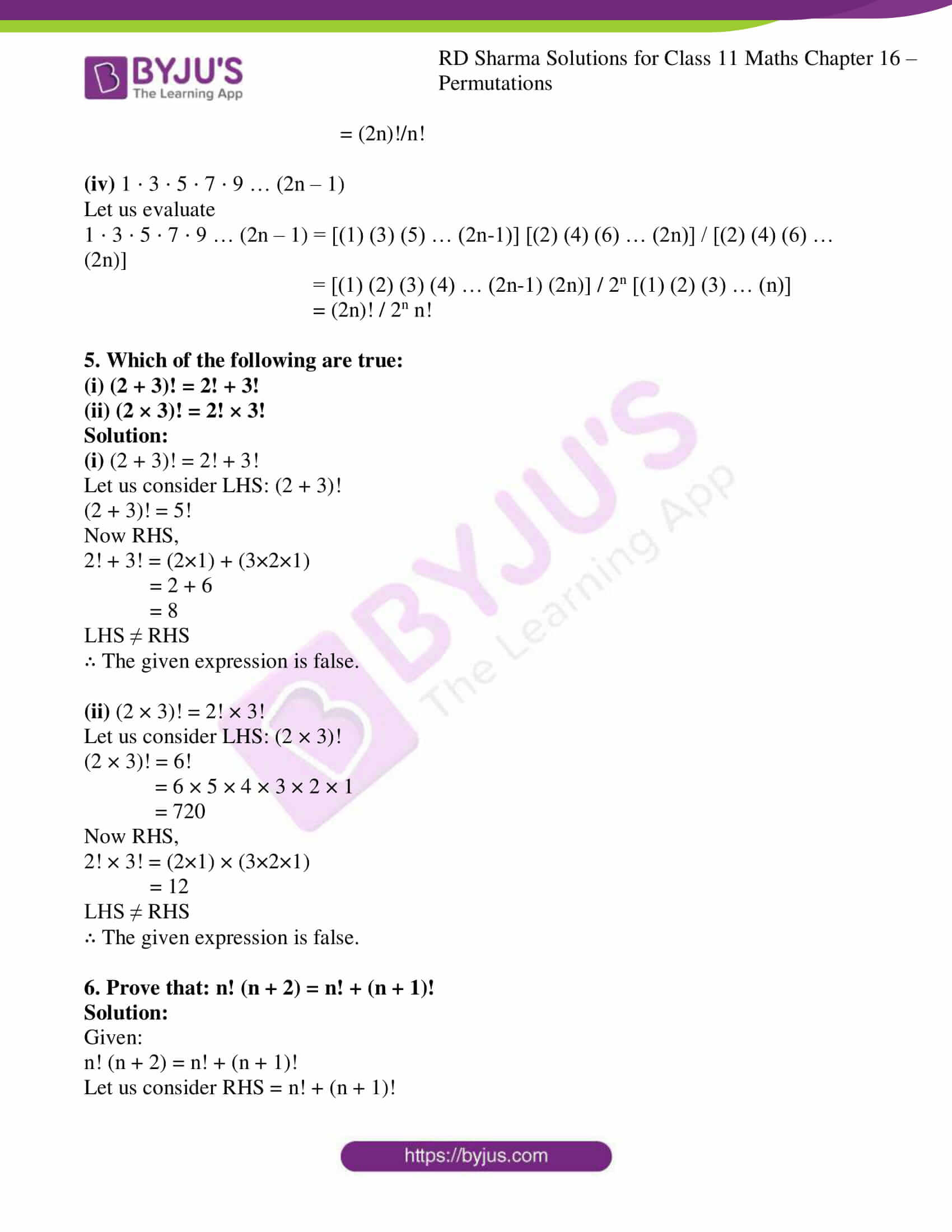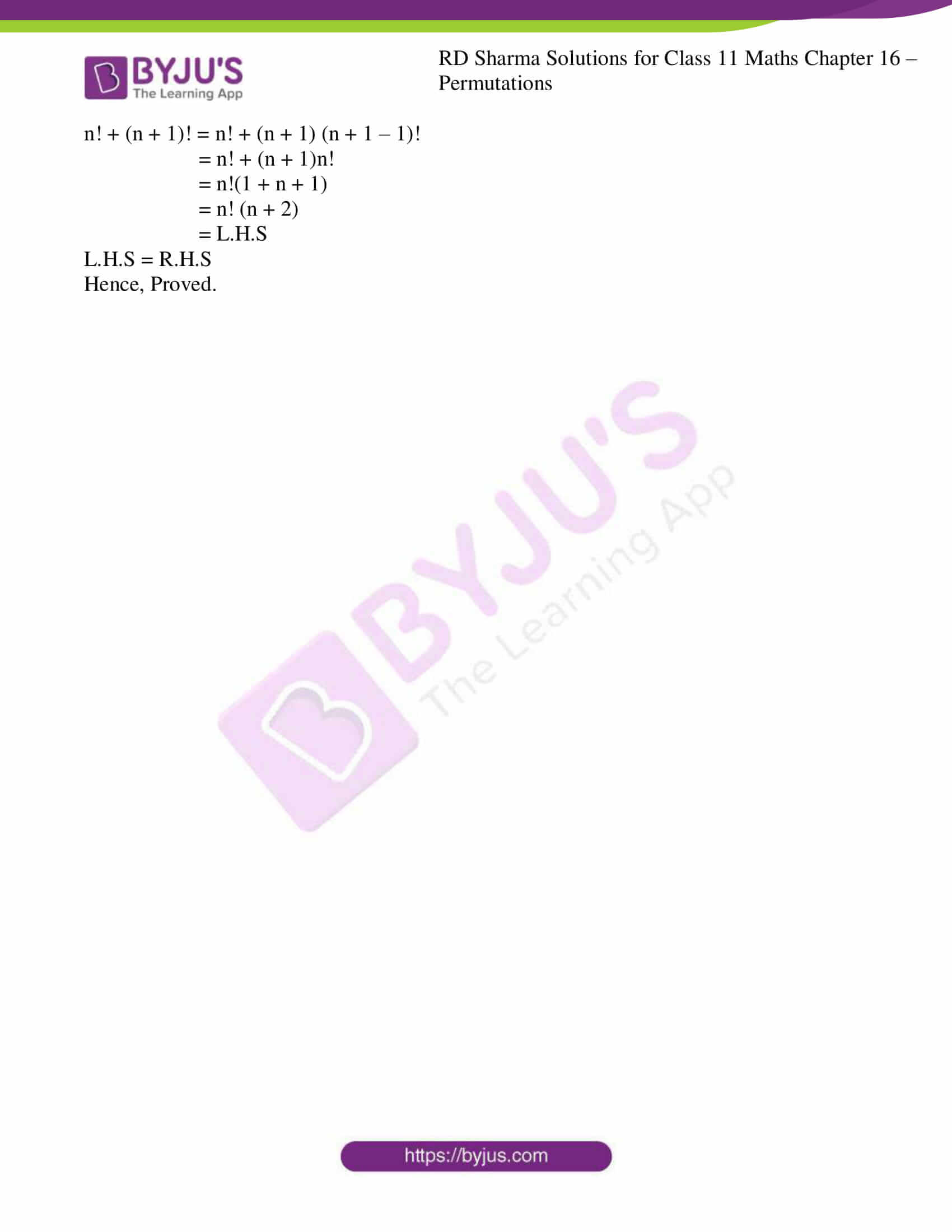### Access answers to RD Sharma Solutions for Class 11 Maths Exercise 16.1 Chapter 16 – Permutations

1. Compute:

(i) 30!/28!

(ii) (11! – 10!)/9!

(iii) L.C.M. (6!, 7!, 8!)

Solution:

(i) 30!/28!

Let us evaluate,

30!/28! = (30 × 29 × 28!)/28!

= 30 × 29

= 870

(ii) (11! – 10!)/9!

Let us evaluate,

We know,

11! = 11 × 10 × 9 × …. × 1

10! = 10 × 9 × 8 × … × 1

9! = 9 × 8 × 7 × … × 1

By using these values we get,

(11! – 10!)/9! = (11 × 10 × 9! – 10 × 9!)/ 9!

= 9! (110 – 10)/9!

= 110 – 10

= 100

(iii) L.C.M. (6!, 7!, 8!)

Let us find the LCM of (6!, 7!, 8!)

We know,

8! = 8 × 7 × 6!

7! = 7 × 6!

6! = 6!

So,

L.C.M. (6!, 7!, 8!) = LCM [8 × 7 × 6!, 7 × 6!, 6!]

= 8 × 7 × 6!

= 8!

2. Prove that: 1/9! + 1/10! + 1/11! = 122/11!

Solution:

Given:

1/9! + 1/10! + 1/11! = 122/11!

Let us consider LHS: 1/9! + 1/10! + 1/11!

1/9! + 1/10! + 1/11! = 1/9! + 1/(10×9!) + 1/(11×10×9!)

= (110 + 11 + 1)/(11 × 10 × 9!)

= 122/11!

= RHS

Hence proved.

3. Find x in each of the following:

(i) 1/4! + 1/5! = x/6!

(ii) x/10! = 1/8! + 1/9!

(iii) 1/6! + 1/7! = x/8!

Solution:

(i) 1/4! + 1/5! = x/6!

We know that

5! = 5 × 4 × 3 × 2 × 1

6! = 6 × 5 × 4 × 3 × 2 × 1

So by using these values,

1/4! + 1/5! = x/6!

1/4! + 1/(5×4!) = x/6!

(5 + 1) / (5×4!) = x/6!

6/5! = x/(6×5!)

x = (6 × 6 × 5!)/5!

= 36

∴ The value of x is 36.

(ii) x/10! = 1/8! + 1/9!

We know that

10! = 10 × 9!

9! = 9 × 8!

So by using these values,

x/10! = 1/8! + 1/9!

x/10! = 1/8! + 1/(9×8!)

x/10! = (9 + 1) / (9×8!)

x/10! = 10/9!

x/(10×9!) = 10/9!

x = (10 × 10 × 9!)/9!

= 10 × 10

= 100

∴ The value of x is 100.

(iii) 1/6! + 1/7! = x/8!

We know that

8! = 8 × 7 × 6!

7! = 7 × 6!

So by using these values,

1/6! + 1/7! = x/8!

1/6! + 1/(7×6!) = x/8!

(1 + 7)/(7×6!) = x/8!

8/7! = x/8!

8/7! = x/(8×7!)

x = (8 × 8 × 7!)/7!

= 8 × 8

= 64

∴ The value of x is 64.

4. Convert the following products into factorials:
(i) 5 ⋅ 6 ⋅ 7 ⋅ 8 ⋅ 9 ⋅ 10

(ii) 3 ⋅ 6 ⋅ 9 ⋅ 12 ⋅ 15 ⋅ 18

(iii) (n + 1) (n + 2) (n + 3) …(2n)
(iv) 1 ⋅ 3 ⋅ 5 ⋅ 7 ⋅ 9 … (2n – 1)

Solution:

(i) 5 ⋅ 6 ⋅ 7 ⋅ 8 ⋅ 9 ⋅ 10

Let us evaluate

We can write it as:

5 ⋅ 6 ⋅ 7 ⋅ 8 ⋅ 9 ⋅ 10 = (1×2×3×4×5×6×7×8×9×10)/(1×2×3×4)

= 10!/4!

(ii) 3 ⋅ 6 ⋅ 9 ⋅ 12 ⋅ 15 ⋅ 18

Let us evaluate

3 ⋅ 6 ⋅ 9 ⋅ 12 ⋅ 15 ⋅ 18 = (3×1) × (3×2) × (3×3) × (3×4) × (3×5) × (3×6)

= 36 (1×2×3×4×5×6)

= 36 (6!)

(iii) (n + 1) (n + 2) (n + 3) … (2n)

Let us evaluate

(n + 1) (n + 2) (n + 3) … (2n) = [(1) (2) (3) .. (n) … (n + 1) (n + 2) (n + 3) … (2n)] / (1) (2) (3) .. (n)

= (2n)!/n!

(iv) 1 ⋅ 3 ⋅ 5 ⋅ 7 ⋅ 9 … (2n – 1)

Let us evaluate

1 ⋅ 3 ⋅ 5 ⋅ 7 ⋅ 9 … (2n – 1) = [(1) (3) (5) … (2n-1)] [(2) (4) (6) … (2n)] / [(2) (4) (6) … (2n)]

= [(1) (2) (3) (4) … (2n-1) (2n)] / 2n [(1) (2) (3) … (n)]

= (2n)! / 2n n!

5. Which of the following are true:

(i) (2 + 3)! = 2! + 3!

(ii) (2 × 3)! = 2! × 3!

Solution:

(i) (2 + 3)! = 2! + 3!

Let us consider LHS: (2 + 3)!

(2 + 3)! = 5!

Now RHS,

2! + 3! = (2×1) + (3×2×1)

= 2 + 6

= 8

LHS ≠ RHS

∴ The given expression is false.

(ii) (2 × 3)! = 2! × 3!

Let us consider LHS: (2 × 3)!

(2 × 3)! = 6!

= 6 × 5 × 4 × 3 × 2 × 1

= 720

Now RHS,

2! × 3! = (2×1) × (3×2×1)

= 12

LHS ≠ RHS

∴ The given expression is false.

6. Prove that: n! (n + 2) = n! + (n + 1)!

Solution:

Given:

n! (n + 2) = n! + (n + 1)!

Let us consider RHS = n! + (n + 1)!

n! + (n + 1)! = n! + (n + 1) (n + 1 – 1)!

= n! + (n + 1)n!

= n!(1 + n + 1)

= n! (n + 2)

= L.H.S

L.H.S = R.H.S

Hence, Proved.